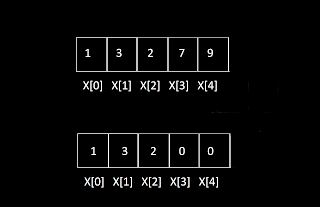### Introduction to Arrays : One Dimensional Array

In this module, we are going to discuss,
• Types of arrays
• The time complexity of basic operations
• Memory allocation of 1 D arrays
• Drawbacks of arrays
Simple variables were used to store a single value of a particular data type
for example int X;

Now suppose we want to store many data items of the same type, in such cases array comes into the picture

An Array is a collection of similar data items that may be of int type, char type, float type, or user-defined types such as structures or class. They are referred by a common name which is the name of an array.

Formal Definition: Contiguous area of memory consisting of equal-size elements indexed by contiguous integers. Let's understand this more deeply through this video from YouTube and then move on with notes.

Types of Arrays :
There are two types of arrays :

#### One dimensional array: It is a collection of similar data items. Multidimensional array: It is a collection of similar data items where each item is an array in itself. For example, a two-dimensional array is a collection of one-dimensional arrays.

One dimensional array
The one-dimensional array is defined by specifying its name, size, and data types of the item.

SyntaxData_type array_name[size];
Exampleint arr;

Initialization : Data_type array_name[size] = { value_1, value_2, ... , value_size };The initial values are enclosed in the { } braces and are separated by a comma.
int X = { 1, 3, 2, 7, 9 };
Since array always starts with 0 indexes. So the first element is represented by X, second element by X, and so on.
int X = { 1, 3, 2 };
Since only three elements of an array X are initialized but the memory is allocated to five elements so the remaining two elements are initialized to zero.

How they are stored in memory?
The array elements are stored in consecutive memory locations and the array elements are accessed by their index value.

array_address + element_size * ( i - first_index)

int X = { 12, 54, 34, 0, -56, 87, 98, 23 };

Let's say array address of X is 1000,
what is the address of the element at index 4?

address of element at index 4 is
1000 + 4 * ( 4 - 0 ) = 1016

Accessing and modifying array elements
The array elements are accessed by using their index, X will retrieve the value stored in the array at index 3.

Let's say initially an array with a capacity of 7 elements have four elements { 5, 8, 3, 12 }

Now we add 4 at the end

Now we remove an element from the end

Now we add 7 to the beginning, for that first we need to shift all elements to the right by one unit and then add 7 at the beginning

Now to remove the element present at the beginning we need to shift all elements except the first element to the left by one unit

Now to insert element 9 after 8, we need to move all elements after 8 to the right by one unit and then place 9 just after 8

Now to remove 8 we need to shift all elements after 8 towards left by one unit

Time Complexities of various operations.
Drawbacks of array
• We need to know the amount of space we are going to use in advance
• The desired amount of contiguous memory may not be available but it may be available in separate locations
• Adding and removing elements in the beginning or middle is linear time.
Practice Problems
1. Monk and Rotation ( HackerEarth )
2. ( HackerEarth )
3. ( HackerEarth )
4. ( HackerEarth )
5. ( HackerEarth )
To see how we use Arrays in solving problems let us solve the above problem Pairs here.
Problem Statement: Find no. of ways of selecting a pair of prime number
and a composite number $\left(q\right)$ in a given range .

Solution: To solve this problem, we will precompute the number of prime and composite numbers in the range up to 100001 and store them in the array.

• prime array will have count of prime number up to index i.
• like prime=0, prime number in the range [0,0] is 0,
• prime=0, prime number in the range [0,1] is 0,
• prime=1, prime number in the range [0,2] is 1 which is 2,
• prime=2, prime number in the range [0,3] is 2 which are 2,3.
• and so on.
• Same for composite array will have count of composite number up to index i.
• Then number of primes, and composite in range l,r would be.,
• primes=prime[r] - prime[l-1];
• composites=composite[r] - composite[l-1];
• Number of ways = primes * composite
• The time complexity of this algorithm is O (n)
Implementation

Summary
• The array is a collection of similar items that are referenced by a common name. The array can be one dimensional or multidimensional.
• An array name always refers to the base address. Array elements are stored in contiguous memory locations.
• constant time to add/remove at the end
• linear time to add/remove at an arbitrary location
In the next module, we will look into multidimensional arrays. If you have any content or any doubt related to this module, mention in the comment section.

1.could you explain a bit more as to how have you come up with those time complexity values?

2.O(1) or constant time means the number of operations are constant and do not depend on the input size of the problem. i e growth rate is independent of the problem size n

O(n) or linear time means the number of operations are proportional to the input data size of the problem, i e growth rate is linearly proportional to the input size of the problem.

1.3.Great initiative!!!